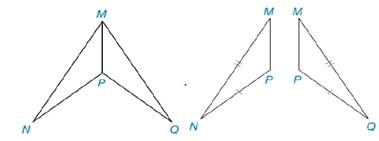Chapter 3.1, Problem 20E### Elementary Geometry for College St...

6th Edition
Daniel C. Alexander + 1 other
ISBN: 9781285195698

#### Solutions

Chapter
Section### Elementary Geometry for College St...

6th Edition
Daniel C. Alexander + 1 other
ISBN: 9781285195698
Textbook Problem
3 views

# In Exercises 19 and 20, the triangles to be proved congruent have been redrawn separately. Congruent parts are marked.a) Name an additional pair of parts that are congruent by using the mason Identity.b) Considering the congruent parts, state the reason why the triangles must be congruent. Δ M N P ≅ Δ M Q PTo determine

a)

To find:

The additional pair of the part that are congruent by using the reason identity.

Explanation

Calculation:

Consider the given diagrams.

Figure (1)

Figure (2)

Since, ΔMNPΔMQP.

In given figure (2), it is clear that the line segments MN¯ and NP

To determine

b)

To find:

The reason for the triangle to be congruent.

### Still sussing out bartleby?

Check out a sample textbook solution.

See a sample solution

#### The Solution to Your Study Problems

Bartleby provides explanations to thousands of textbook problems written by our experts, many with advanced degrees!

Get Started

#### Find more solutions based on key concepts